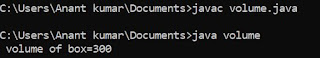## Thursday, 12 July 2018

### Program to calculate sub of two matrix of order m * n in C using for loop statement

/* subtraction  of matrix */
#include<stdio.h>
void main ()
{
int  ,  ,  ;
int  r1 , r2 , c1 , c2 ;
int i , j ;
clrscr () ;
printf ("\n Enter the no of rows for first element:") ;
scanf ("%d", &r1) ;
printf ("\n Enter the no of column for first element:") ;
scanf ("%d", &c1) ;
printf ("\n Enter the no of rows for second element:") ;
scanf ("%d", &r2) ;
printf ("\n Enter the no of column for second element:") ;
scanf ("%d", &c2) ;
if ( ( r1!=r2) && (c1!=c2) )
{
printf ("\n subtraction is not possible:") ;
getch () ;
exit () ;
}
for ( i = 0 ; i < r1 ; i++ )                   /* Input of first matrix */
{
for ( j = 0 ; j < c1 ; j++ )
{
printf ("\n Enter a number:") ;
scanf ("%d", & a {i}{j}) ;
}
}
for ( i = 0 ; i < r2 ; i++ )                    /* Input of second matrix */
{
for ( j = 0 ; j < c2 ; j++ )
{
printf ("\n Enter a number:") ;
scanf ("%d", & b {i}{j}) ;
}
}
for ( i = 0 ; i < r1 ; i++ )             /* print first matrix */
{
for ( j = 0 ; j < c1 ; j++ )
printf ("%3 d", a {i}{j}) ;
printf ("\n") ;
}
for ( i = 0 ; i < r2 ; i++ )              /* print second matrix */
{
for ( j = 0 ; j < c2 ; j++ )
printf ("%3 d", b{i}{j}) ;
printf ("\n") ;
}
for ( i = o ; i < r1 ; i++ )                   /* calculation of matrix */
{
for ( j = 0 ; j < c1 ; j++ )
{
c {i}{j} = a {i}{j} - b {i}{j} ;
}
}
for ( i = 0 ; i < r1; i++ )
{
for ( j = 0 ; j < c1: j++ )
printf ("%3 d", c {i}{j}) ;
printf ("\n") ;
}
getch () ;
}

### Topics used :

In C programming array is very important topic as we discuss earlier there is two types of array in c you may read at https://www.programmingponds.com/2018/07/array-in-c.html and at https://www.programmingponds.com/2018/07/c-array-types.html .

### How to subtract two matrix :

As we know that for subtract of matrix possible there must be number of rows of first matrix should be equal to the number of rows of second matrix and the number of column of first matrix is also equal to the number of column of second matrix for this we have to apply a conditional statement .